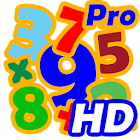Math Quiz HD Pro

All Android applications categories

All Android games categories# Math Quiz HD Pro

0 0

## Screenshots

Description

Math Quiz HD based on the four basic arithmetic addition, subtraction, multiplication and division, and helps you to train your brain in a funny way.

It is suitable for all ages over 12. The degree of difficulty increases with each level.

Features:
- 6 "standard game modes" (addition, subtraction, multiplication, division, addition/subtraction and "mix" with all four basic arithmetic
- special MathPuzzle-Mode
- suitable for all ages from 12
- nice HD graphic
- …

The "MathQuiz HD Pro" version is identical to this version, however, contains no advertising and is a little recognition for my work.

Tags: math quiz , question wallpaper maths , math question wallpapers , mathematical question wallpaper , quiz wallpapers hd , math quiz question wallpaper , mathematical quiz , mathematical questions with images , quiz wallpaper funny images